Calculator
from KEUWLSOFT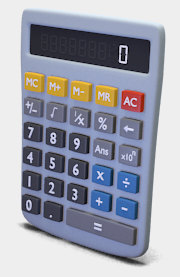Calculator User Guide

This is a basic calculator which operates and looks much like a stand alone one. It has a 9 digits main display and two extra for the x10 exponent. It can handle numbers as large as 9.99999999E+99 and as small as 1.00000000E-99. It has:
• A typical number pad with x ÷ + - operators and a large equals button.
• Memory buttons for storing and recalling values.
• Square root, Reciprocal and Percent.
• Negate, Undo, Ans and x10n to make number entry easier.

Most of these will now be explained along with other features/settings available.

Operators

Multiply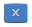, Divide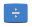, Addand Subtract. Type in the first number, type in the operator and then the second number. Finally press equals to get the result. Longer sums can be strung together as each further press of an operator will use the result of the previous operator operated on the two numbers as the first number in the next operation.

A few examples: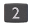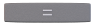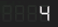.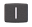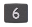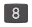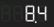..
Remember the the operators are applied in order. The result of the previous operator is shown as soon as the next operator is pressed on the calculator. i.e. it functions just like an typical hand held calculator. If the sum was written out on paper, for example, and treated as an expression, there is an order in which the operators should be handled. However, this ordering is ignored for most basic hand held calculators since they only ever see two operands at a time, calculating as they go along.

Memory Functions

The four orange memory functions allow you to add subtract, clear or recall a value.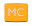Clear the memory value back to zero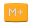Adds the currently displayed value to the memory.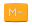Subtracts the currently displayed value from the memory.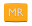Recalls the memory value and shows it in the display.

Example: If you want to store the currently displayed value to memory, pressto clear the memory and thento add the current value.

Function Buttons

Three function buttons are included on this calculator. These will affect the current number according to the mathematical function. They are now explained:Calculates the square root of the current number. If no number has been entered yet, a square root flag is shown top left of the display and the square root operation is done after the number has been entered.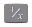Computes the reciprocal of the current number. The reciprocal is just 1 divided by the number.The percent button assumes the second number just entered is a percentage of the previous number and applies the operator which was used.

Example 1, An awesome jacket, originally at 52.90, is on sale with 30% off. How much does it cost? To do this we could type the following sequence: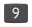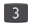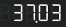.

Example 2, There are 1000 rabbits in a field, they increase by 80% in the first year and by 50% in the second year. how may rabbits? To do this we could type the following sequence:.
To write it out, we have... 1000 plus 80% of 1000 is 1800. 1800 plus 50% of 1800 = 2700.

Note that whilst most calculators will treat % as being a percentage of the previous number. Some calculators will just convert the percentage to a fraction by dividing it by 100. Some calculators will mix methods depending on the operator. We stick to the first method in this app. Thus, for example 20 + 50% is read as 20 plus 50% of 20 = 30 (not 20 plus 50/100 = 20.5) and similarly 20 x 50% is read as 20 times 50% of 20 = 200 (not 20 times 50/100 = 10). If this seems a little strange or counter intuitive, you might be an scientist or engineer, in which case you will probably be better just ignoring percent buttons anyway.

Manipulate Number Buttons

Three are buttons that make number entry easier.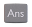This recalls the previous answer which is stored each time equals is pressed.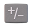This negates the current number. Use this button to enter negative numbers.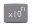Whilst entering in a number, pressing this will allow the x10 exponent to be entered or negated instead. Pressing it again will toggle back to entering the main number.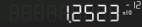Exponential notation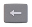Undo the last number (or point) entered. This will only work on numbers that can be edited. Once an operation or function has been applied, the number can no longer be modified.

Settings

By clicking on the settings icon at the top left of the screen we enter the settings menu, with some more options:

Sound On - Turn sound on or off.

Vibrate - Vibrate when counting.

Stay Awake - Stops your device from sleeping whilst the app is running.

Always show values less than 1 using Exponential notation - Some users will not be familiar with exponential(scientific) notation, so the default is to show numbers less than one by writing them out. For example 1.41421356E-05 in scientific notation would be shown as 0.00001412. Since it has fewer significant figures, using scientific is better if you are familiar with it.

and...

The values stored in Ans and Mem are retained for the next time you run the app.

Long pressing on the display will copy that value in scientific format to the clipboard so that it can be pasted into another application.

Not all calculators are made equal. Be aware that functionality can vary. If unsure, check for whichever calculator you are using, that it operates the way you expect for the type of calculation you are doing first.